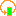#PReMo -- Probabilistic Recursive Models analyzer

This is the homepage of a tool called PReMo (read as primo). PReMo is capable of analyzing:

• Recursive Markov Chains (RMCs, equivalent to probabilistic Pushdown Systems and Tree-like QBDs)
• Recursive Simple Stochastic Games (RSSGs, also with arbitrary positive rewards)
• discrete-time Quasi-Birth-Death processes (QBDs, equivalent to probabilistic 1-counter automata)
• Stochastic Context Free Grammars (SCFGs)
• fixed point solutions of arbitrary equation systems (without any guarantee of termination)
RMC is a natural model for recursive imperative programs with probabilistic transitions. Probability comes either from an explicit randomization like in the Quicksort algorithm or by abstracting some aspects of a program.

### What's new

Current version of PReMo is 1.3.1 For the changes see the changelog.

### Installation

If that does not work, one can always run is from the command-line like this:
`	java -jar PReMo-<version>-<your OS name><arch>.jar`
If you encounter any problems when running PReMo first see the troubleshooting notes and then write an email to the address given at the bottom of this web page.

### Examples

• Equations systems generated for large Stochastic Context Free Grammars(SCFGs) used in Natural Language Processing(NLP): grammars
(The number of production for a single non-terminal can be as huge as few thousands and as a result the expression defining such non-terminal is very long and very hard to manipulate. That's why I've split all expressions in a chunks of 100 and did variable chaining, where each such a variable is a sum of new variables corresponding to some subexpression of the expression originally defining that variable. Names of the main variables, in which termination probability we are really interested in, are n_number.)
• Some small examples of RMCs, RSSGs, SCFGs and equation systems: small examples
• Small example QBDs specified as p1CAs: QBDs
• A big example of a random RMC with 10 000 variables: big example
• An RMC model of randomized Quicksort algorithm sorting an array of size 7: quicksort
To run any of the examples:
1. Unzip them somewhere.
2. Run PReMo as described above.
3. Choose from the File menu Open -> RMC, RSSG, SCFG, QBD, Equation depending on what type of an example you would like to run.
4. Then from the Run menu Parse
5. after parsing successfully choose Generate equations -> termination probability
6. and finally choose the solver you would like to use from the Find solution submenu

### Documentation

The documentation is available here:
PDF format   and   HTML format (unfortunately some of the pictures are missing)

### Coming up in PReMo

• documentation for the 1.2 and 1.3 version of PReMo
• high level input model specification language for PReMo that allows the user to use variables, arrays, while loops etc.
• reachability and safety analysis
• expected and average reward computation for multi-exit RMCs
• stopping, pausing and resuming solver's computations
• same more examples, especially for 1-RSSGs

` d.wojtczak -- at -- liverpool.ac.uk `